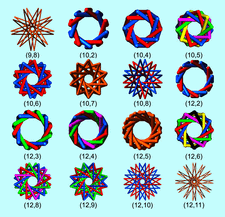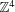# issue contentsFOUNDATIONSADVANCES
ISSN: 2053-2733

# September 2020 issueCover illustration: Weavings, knots, links and related geometrical structures, such as polycatenanes, are currently of considerable interest in materials chemistry. In particular, reticular chemistry is concerned with the directed assembly of symmetrical frameworks from molecular components. The cover image shows isogonal torus knots (orange) and links (multicoloured), (p,q), for p = 9-12 [O'Keeffe & Treacy (2020). Acta Cryst. A76, 611-621]. There is one kind of vertex (isogonal) and two kinds of stick in each. These are shown for the maximum-girth embedding, where girth is defined as the ratio of the shortest stick separation divided by the longer stick length.

## scientific commentaries

### Quaternions: what are they, and why do we need to know?

The significance of the work by A. J. Hanson [Acta Cryst. (2020), A76, 432–457] on finding the optimal alignment of pairs of spatial and/or orientation data sets is discussed.

## topical reviews

### Inflation versus projection sets in aperiodic systems: the role of the window in averaging and diffraction

Averaged quantities such as mean shelling numbers, scaling behaviour or diffraction for cut-and-project sets can conveniently be computed in internal space, also for systems with fractally bounded windows.

## research papers

### Embedding-theory-based simulations using experimental electron densities for the environment

For the first time, the use of experimentally derived molecular electron densities as ρB(r) in calculations based on frozen-density embedding theory (FDET) of environment-induced shifts of electronic excitations for chromophores in clusters is demonstrated. ρB(r) was derived from X-ray restrained molecular wavefunctions of glycylglycine to obtain environment densities for simulating electronic excitations in clusters.

## research papers

### Multiplicity-weighted Euler's formula for symmetrically arranged space-filling polyhedra

For many tested cases of identical space-filling polyhedra, such as the space-group-specific asymmetric units or Dirichlet domains, the numbers of their faces (Fn), edges (En) and vertices (Vn), in each case normalized by division by the multiplicity of their (potentially special) symmetry position, fulfill a modified Euler's formula FnEn + Vn = 1.

### On Cayley graphs ofCayley graphs ofwith valency 10 have been enumerated which correspond to generating sets of integral vectors with components −1, 0, 1 and which are embedded in a four-dimensional Euclidean space without edge intersections.

### X-ray scattering study of water confined in bioactive glasses: experimental and simulated pair distribution function

Water confined in bioactive glasses is studied by total X-ray scattering. Three structural configurations can be distinguished, from bulk-like water in the pore centre to a strongly distorted layer on the pore surface.

### Pure discrete spectrum and regular model sets in d-dimensional unimodular substitution tilings

The equivalence between pure discrete spectrum and regular model sets in d-dimensional unimodular substitution tilings is discussed.

### Isogonal weavings on the sphere: knots, links, polycatenanes

Non-periodic knotted and linked structures that can be built using one kind of vertex (isogonal) and two kinds of edge (stick) are described. The major types of these structures are identified and reported with optimal embeddings.

### On the Σ classes in E6

The cone of positive definite quadratic forms is subdivided. The equivalence classes are determined for several levels.

## short communications

### Tilings by hexagonal prisms and embeddings into primitive cubic networks

A tiling (not necessarily face-to-face) of the 3D Euclidian space by hexagonal prisms can be embedded into the primitive cubic net of either three or four dimensions.
Follow Acta Cryst. A